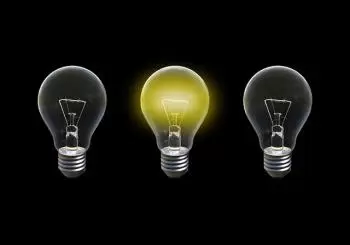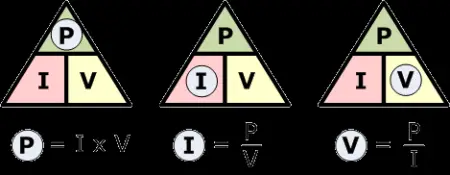# Watt's Law: Formula, Relationship with Ohm's Law and UseWatt's law defines the relationship between power, amperage, and voltage in an electrical circuit. Watt's law states that: "the power of an electrical circuit or device is the product of its voltage and the current flowing through it."

The electric potential determines the electrical energy consumption of a circuit. In turn, the power required depends on the devices' resistance, input voltage, and the current flowing through the resistance.

The law was developed by James Watt (1736-1819), a Scottish engineer and inventor. Watt is also credited with inventing the concept of horsepower. A horsepower is a unit of work or the rate at which work is done.

## Watt's Law Formula

Watt's law formula to calculate the power of an electrical resistor is presented as follows:

P = V·I

Where

• “P” is the electrical power of an electrical resistor measured in watts (W).

• “V” is the voltage applied to the resistance of a circuit expressed in volts (V).

• “I” is the amperage of the electric charge measured in amps (A).

This formula can verify that the power consumed is directly proportional to the difference in input and output voltage and current intensity.

There are three possible formulas for calculating electrical power in a circuit. In order to remember them, we can use the following triangle's rule.## What Is the Relationship Between Watt's Law and Ohm's Law?

Watt's law relates power to voltage and current intensity. On the other hand, Ohm's law relates the voltage to the current intensity and the electrical resistance of a circuit.

Ohm's law formula is:

V = I · R

Where

• “V” is the voltage expressed in volts (V).

• “I” is the amperage expressed in amperes (A).

• “R” is the electrical resistance offered by a circuit measured in ohms (Ω).

Since both laws use the same electrical quantities, they are often combined to get some useful equations.

As we can deduce from the formula, the voltage drop with the amperage and the resistance.

## Uses and Applications of Watt's Law

Some of the applications of Watt's law include:

• Measurement of the actual amount of energy that an electric generator can produce.

• Measurement of the electrical power that a building can consume.

• If the power and voltage of the electrical component are known, the amperage can be measured. On the other hand, the voltage can be obtained if the power and current intensity are known.

• The formulas obtained from the combination of Watt's law and Ohm's law can be applied to determine the electrical resistance of an electrical component.

• Definition of the minimum cross-sectional area of an electrical wire according to the power requirement of a light bulb.

## Who Was James Watt?

James Watt was a Scottish inventor and mechanical engineer who improved the Newcomen steam engine. He is credited with developing the concept of horsepower and his improvements to the steam engine. His improvements led to the widespread adoption of the steam machine in industry and commerce.

Watt's most important contribution was his development of an improved steam engine, which was more efficient than the existing Newcomen engines. He called his machine the "separate condenser" because it had a separate chamber for condensing steam. Therefore, the cylinders could be kept hot, vastly increasing their efficiency.

Author:

Published: December 16, 2021
Last review: December 15, 2021